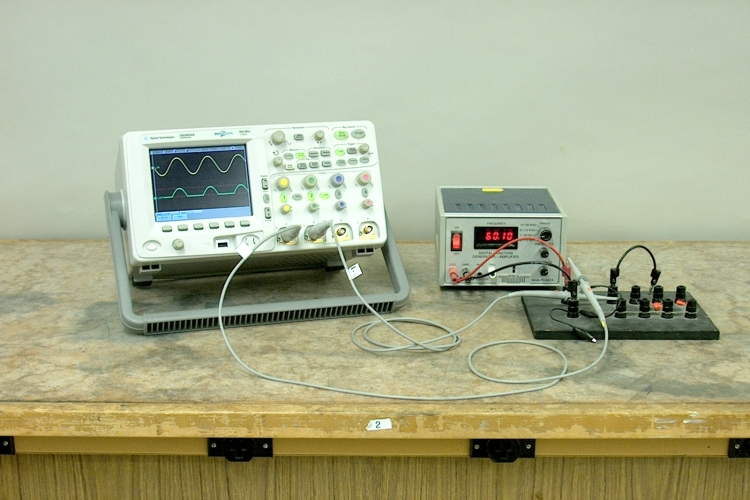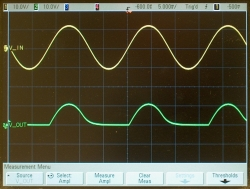A diode is a device that conducts electricity in one direction, but not the opposite direction. (See demonstration 64.56 -- Light-emitting diode (LED).) If one tries to pass an AC current through a diode, it will therefore conduct only during one half-cycle, the cycle during which it is forward biased. The simplest type of rectifier circuit is a diode with a current-limiting (or load) resistor in series with it. In this demonstration, the anode of a 1N914 (silicon switching) diode is connected to the output of a signal generator, and the cathode is connected via a 100-kilohm resistor to ground. (Please note: The 1N914 is NOT typically used in power rectifier circuits. It is, however, more than capable of handling the currents and voltages used in this demonstration (vide infra).) The top trace of the oscilloscope shows the voltage at the output of the signal generator (at the anode of the diode), and the bottom trace shows the voltage across the resistor. As is visible in the photograph above, the diode conducts during one half-cycle, and during the other half-cycle, the voltage across the resistor is zero. The diode does not begin to conduct, however, until the applied voltage exceeds the junction potential, so the half-cycles in the bottom trace are slightly narrower than the distance between zero crossings in the top trace. The amplitude measurements in the enlarged photograph below make this clear:The peak-to-peak voltage of the input signal is 23.8 volts. Half this would be 11.9 volts, but the measured amplitude of the bottom trace is 11.6 volts, about 0.3 volts lower.

In order to change the rectified voltage from a pulsating DC voltage to a steady one, we can place a capacitor across the load. The capacitor charges during the positive-going side of each positive half-cycle, and it discharges through the load during the rest of the cycle. Depending on the RC time constant (see demonstrations 64.51 -- RC circuit to galvanometer and 64.54 -- RC circuit to oscilloscope), the discharge takes more or less of the time between the peaks, and the filtering action flattens the output voltage to a greater or lesser extent. The circuit board has three capacitors, one end of each connected to ground and the other end free, and a jumper with which you can select one of them to put in parallel with the load resistor. The three photographs below show the result of adding each of these capacitors:0.01 μf 0.1 μf 10 μf

We see that the filtering afforded by the 0.01-μf capacitor raises the tail of the half-cycle, the 0.1-μf capacitor smooths the waveform even more, leaving a large ripple, and the 10-μf capacitor takes out enough of the ripple to make the DC voltage essentially flat.

For a given rectifier circuit, the size of the ripple can be expressed as the “ripple factor,” r = Iac/Idc = Vac/Vdc. r also equals 1/(2√3fCRL), where f is the frequency of the ac component. As we can see from the traces above, for the half-wave rectifier the frequency equals that of the input voltage. This expression holds only for wave forms that are approximately sinusoidal, and for loads that do not cause the output voltage to drop more than about 20% from the peak voltage. Alternatively, we can derive a simple expression for the ripple voltage. If the ripple is small, we can assume that the current through the load is constant, and from I = C(dV/dt) we have ΔV = (I/Ct. Δt is just the period of the ripple, so it equals 1/f, making the expression ΔV = (Iload/fC). In doing this, we have assumed a linear discharge of the capacitor, instead of an exponential, but for constant load current, the discharge is linear. We can see from both of these expressions that for a given frequency, increasing the capacitance reduces the magnitude of the ripple, and whatever the capacitance, increasing the frequency of oscillation of the input voltage also decreases the size of the ripple. We can also see from the first two traces above that as the ripple becomes smaller, the discharge gets closer to being linear.

In this demonstration, not only can you change the value of the capacitor, as shown above, but you can also vary the frequency of the function generator to show its effect on the ripple.

In building a rectifier circuit, one must choose a diode that can safely withstand the current the circuit will have to provide, and also the reverse bias voltage that will be applied to it. Diodes are rated for maximum average forward current, which, since the diode conducts only half the time (positive-going half-cycles only), is roughly 1/2(Vav/RL), where Vav is the average voltage and RL is the load resistance. The peak inverse voltage (PIV), or maximum repetitive reverse voltage (VRRM) is the maximum reverse bias that the diode can withstand. For the unfiltered rectifier, this is just the peak voltage, which in the example shown above is about 12 volts. If one adds a capacitor to filter the output, the PIV doubles to 24 volts, because the capacitor now holds the output at +12 volts while the voltage on the anode of the diode swings to -12 volts. The 1N914 diode used in this demonstration is rated for a VRRM of 100 volts and an average rectified forward current of 200 mA. While these values, especially the average forward current, make this diode unsuitable for use in most power supply applications, this component can easily handle the PIV of 24 volts and average forward current of 0.12 mA present in this demonstration.

References:

1) Howard V. Malmstadt, Christie G. Enke and Stanley R. Crouch. Electronics and Instrumentation for Scientists (Menlo Park, California: The Benjamin/Cummings Publishing Company, Inc., 1981), pp.57-58, 61-62.
2) Paul Horowitz and Winfield Hill. The Art of Electronics, Second Edition (New York: Cambridge University Press, 1994), pp. 45-46, 329-330.
3) Fairchild Semiconductor Corporation. Data Sheet for 1N/FDLL 914/A/B / 916/A/B / 4148 / 4448 Small Signal Diode (2002).